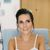# QlikView App Dev

Discussion Board for collaboration related to QlikView App Development.

Announcements
cancel
Showing results for
Did you mean:Creator III

## expression effectively

hi,

i have this expression-

if((sum(amount)-sum(budget))/number <10,'red',

if((sum(amount)-sum(budget))/number <30,'yellow',

if((sum(amount)-sum(budget))/number <50,'green',

if((sum(amount)-sum(budget))/number <70,'blue',

if((sum(amount)-sum(budget))/number <100,'purple')))))

The calculation time of the expression is very long and therefore I wanted to know whether it is possible to write the expression more effectively...

Because the expression (sum(amount)-sum(budget))/number) in each of the if conditions is the same

(something like Match in string)

can someone help?

3 RepliesPartner

You could always do:

=Pick(Floor(

((sum(amount)-sum(budget))/number)

/10)+1,'red','yellow','yellow','green','green','blue','blue','purple','purple','purple')

This doesn't account for negative values so it needs more fine-tuning I guess....Creator III
Author

its not always interval of 10...Creator III
Author

someone have an idea?